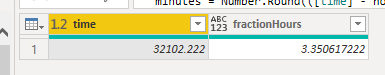cancel
Showing results for
Did you mean:Frequent Visitor

## How do I convert time from 32102,222 to real decimal number

I use data from a database with price and hours.

for instance: price is 27,14 hours are 3h21min2,222sec

In power bi: 27,14 and 32102,222

How do I get the time in decimal number like this? : 3,35061722

1 ACCEPTED SOLUTIONSuper User

Hi @JoseHop ,

*EDIT* Having looked at your previous post, I think this should paste straight into your query as a new custom column:

``````let
hours = Number.Round([PRIJS_PERIODE.PRODUCT_KIND_VAST.DINSDAG_UREN] / 10000),
minutes = Number.Round(([PRIJS_PERIODE.PRODUCT_KIND_VAST.DINSDAG_UREN] - hours * 10000) / 100),
seconds = [PRIJS_PERIODE.PRODUCT_KIND_VAST.DINSDAG_UREN] - (hours * 10000) - (minutes * 100)
in
hours + (minutes * 60 + seconds) / 3600)``````

No, I was after a visual of what the values actually look like in PQ. It's fine though, not a problem.

Add this in a new custom column, assuming your original value is in a column called [time]:

``````let
hours = Number.Round([time] / 10000),
minutes = Number.Round(([time] - hours * 10000) / 100),
seconds = [time] - (hours * 10000) - (minutes * 100)
in
hours + (minutes * 60 + seconds) / 3600)``````

This gives me the following output:Pete

Now accepting Kudos! If my post helped you, why not give it a thumbs-up?

Proud to be a Datanaut!

6 REPLIES 6Memorable Member

``````let
hrs = Number.IntegerDivide([Hours],10000),
mins = Number.IntegerDivide([Hours] - hrs * 10000,100),
secs = Number.Mod([Hours], 100)
in Duration.TotalHours(#duration(0,hrs, mins, secs))``````

To Reproduce:

``````let
Source = Table.FromRows(Json.Document(Binary.Decompress(Binary.FromText("i45WMjLXMTRR0lEyNjI0MNIxMjJSio0FAA==", BinaryEncoding.Base64), Compression.Deflate)), let _t = ((type nullable text) meta [Serialized.Text = true]) in type table [Price = _t, Hours = _t]),
#"Changed Type" = Table.TransformColumnTypes(Source,{{"Price", Currency.Type}, {"Hours", type number}},"en-DE"),
let
hrs = Number.IntegerDivide([Hours],10000),
mins = Number.IntegerDivide([Hours] - hrs * 10000,100),
secs = Number.Mod([Hours], 100)
in
Duration.TotalHours(#duration(0,hrs, mins, secs)))
inFrequent Visitor

hi ronrsnfld, thnx for your answer. The formula looks oke but when I add this then I will get an error.

Probalby I do something wrong:Memorable Member

It appears you do not have a column named Hours.  Since you posted no data, I used obvious column names. You will need to change it to the actual columnFrequent Visitor

Hi Pete, thnx voor your quick response.

I hope I understand your question.

Is this what you are looking for?:

= Table.TransformColumnTypes(Bron,{{"PRIJS_PERIODE.PRODUCT_KIND_VAST.DINSDAG_UREN", type number}})Super User

Hi @JoseHop ,

*EDIT* Having looked at your previous post, I think this should paste straight into your query as a new custom column:

``````let
hours = Number.Round([PRIJS_PERIODE.PRODUCT_KIND_VAST.DINSDAG_UREN] / 10000),
minutes = Number.Round(([PRIJS_PERIODE.PRODUCT_KIND_VAST.DINSDAG_UREN] - hours * 10000) / 100),
seconds = [PRIJS_PERIODE.PRODUCT_KIND_VAST.DINSDAG_UREN] - (hours * 10000) - (minutes * 100)
in
hours + (minutes * 60 + seconds) / 3600)``````

No, I was after a visual of what the values actually look like in PQ. It's fine though, not a problem.

Add this in a new custom column, assuming your original value is in a column called [time]:

``````let
hours = Number.Round([time] / 10000),
minutes = Number.Round(([time] - hours * 10000) / 100),
seconds = [time] - (hours * 10000) - (minutes * 100)
in
hours + (minutes * 60 + seconds) / 3600)``````

This gives me the following output:Pete

Now accepting Kudos! If my post helped you, why not give it a thumbs-up?

Proud to be a Datanaut!Super User

Hi @JoseHop ,

Can you provide a visual example of what your original format looks like and exactly how it looks in PQ, including data types please?

Even better, if you can provide an example of your data by using the 'Enter Data' function and pasting the M code here, we can get you a solution even faster.

Pete

Now accepting Kudos! If my post helped you, why not give it a thumbs-up?

Proud to be a Datanaut!